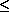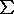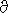•# Statistical descriptors in crystallography

## Uncertainty of measurement

The uncertainty in the result of a measurement, or the uncertainty of the measured value of the specific quantity subject to measurement (i.e. the measurand), expresses doubt about how well the result represents the value of the measurand. The measurement only provides an estimate of the value of a measurand. Since the value of a measurand is an unknowable quantity, its deviation from the measurement result (error) is also unknowable. The uncertainty reflects the lack of exact knowledge of the value of the measurand owing to random and systematic effects, including deficiencies in the model that relates the observations to the measurand. Uncertainty is itself an estimate based on recognized, i.e. known, sources of uncertainty consistent with presently available knowledge; unrecognized, i.e. unknown, sources of uncertainty cannot be taken into account.

Whereas uncertainty designates a general concept, its quantitative measure is called standard uncertainty. The standard uncertainty is an estimate of the standard deviation, i.e. the positive square root of the variance, of the probability distribution of the possible values of the measurand. The standard uncertainty may consist of several components corresponding to different sources of uncertainty. The term standard uncertainty (s.u.) is synonymous with, and replaces, the familiar term estimated standard deviation (e.s.d.) in statements of the statistical uncertainties of data and measurement results. Uncertainty components may be classified into two categories based on their method of evaluation, known as type A and type B. The purpose of this classification is to indicate two fundamentally different methods of evaluating uncertainty components. This contrasts with the traditional classification of uncertainty as arising from a combination of random and systematic effects. Categorizing the methods of evaluating uncertainty components rather than the components themselves avoids the traditional ambiguities associated with attempts at distinguishing between random and systematic effects. The result of a type A evaluation of an uncertainty component may be referred to as a type A standard uncertainty, that of a type B evaluation as a type B standard uncertainty. Both types represent standard deviations.

In crystallography as elsewhere, the measurands Y are usually derived from a number of other observed quantities X1, X2, ... , XN, each of which is also a source of uncertainty:

(W1) . . Y = f (X1, X2, ..., XN).

The functional relationship f is generally non-linear. Measurands of interest to structural crystallographers include atomic coordinates, bond lengths and displacement tensors; the quantities Xn include diffraction intensities. The standard uncertainty of a derived quantity Y is called the combined standard uncertainty (c.s.u.). This term should be used only where it is essential to distinguish it from the component standard uncertainties of the quantities Xn. The combined standard uncertainty of the estimate y of Y is calculated according to (W2) from the individual uncertainty components u(xn) of the estimates xn of Xn, obtained from both type A and type B evaluations. The coefficients of the estimated variances u2(xn) and covariances u(xm)u(x n)r(xm, xn) are obtained by linearizing f (X1, ..., XN) in the vicinity of the xn (1nN) with a first-order Taylor series, leading to the usual approximation:

(W2) . . uc2(y) =n=1N (f /Xn)2u2(x n)
+ 2(f /Xm)(f /Xn)u(xm) u(xn) r(xm,xn),

where r(xm,xn) is the correlation coefficient of xm and xn. Equation (W2) is the law of propagation of uncertainty. It shows how the combined standard uncertainty of the measurement result is obtained from the standard uncertainties of the quantities upon which it depends. The calculation of standard uncertainties of measurands refined by least squares is described in the section on refinement.

In the simplest case, a type A standard uncertainty is calculated from a series of N observations; it is the familiar standard deviation of the mean of the N observations, i.e. N times the positive square root of the sample estimate of the population variance. More generally, any estimate of uncertainty based on a statistical analysis of experimental data is of type A. If the probability density function (p.d.f.) of the measurand is sufficiently well known, as is the case for counting statistics represented by the Poisson distribution, the standard uncertainty may be derived from very few or even one single observation and is also classified as type A. Even though net diffraction intensities do not obey Poisson statistics (Wilson, 1992), their (combined) standard uncertainties from counting statistics are calculated from those of the peak and background counts which in turn are estimated from Poisson statistics. It is good practice to derive scaling factors of intensities correcting for crystal decay as well as additional contributions to the uncertainty from the variations of periodically measured check reflections, i.e. of additional series of repeated observations. Corrections for anisotropic radiation damage have been described by Abrahams & Marsh (1987). Uncertainties of diffraction intensities derived by such widely applied procedures are thus of type A. Uncertainties of the results of a structure determination derived by proper statistical procedures, usually from a least-squares refinement on intensities, are also of type A.

A type B standard uncertainty is obtained by means other than the statistical analysis of observations. Essentially, it reflects subjective opinion or a priori information on the uncertainty of the result of a measurement: it is evaluated by scientific judgement using all relevant information on the possible variability of an observation. The pool of information may include previous observations, experience with or general knowledge of the behaviour and properties of relevant materials and instruments, manufacturer's specifications, data provided in calibration and other certificates, and uncertainties assigned to reference data taken from handbooks. In a structure refinement, uncertainties assigned to restraints of distances, angles and vibrationally rigid bonds are of type B unless they are derived from sample variances of relevant quantities observed in published structures. Most uncertainty components in the diffraction intensities are of type A, although it may be possible on the basis of experience to recognize effects for which the associated uncertainties are obtained from type B evaluations: thus a type B component may allow for doubts concerning, for example, the estimated shape and dimensions of the diffracting crystal and the subsequent corrections made for absorption, the reliability of an extinction correction, etc.

[Next], [Previous]

© 1989, 1995 International Union of Crystallography
Updated 23rd Sept. 1996

These pages are maintained by the Commission Last updated: 15 Oct 2021